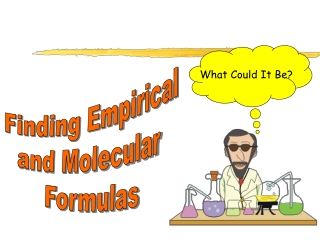DownloadDownload PresentationWhat Could It Be?

# What Could It Be?

Download Presentation## What Could It Be?

- - - - - - - - - - - - - - - - - - - - - - - - - - - E N D - - - - - - - - - - - - - - - - - - - - - - - - - - -
##### Presentation Transcript

1. What Could It Be? Finding Empirical and Molecular Formulas

2. Empirical Formulas The empirical formula is the simplest whole number ratio of the atoms of each element in a compound. Note: it is not necessarily the true formula of the compound. Example: The molecular formula for glucose is C6H12O6, but its empirical formula is CH2O It’s kind of like a fraction reduced to its lowest terms.

3. Molecular Formulas The molecular formula gives the actual numbers of each element, and thereby represents the true formula of the compound.

4. Calculating the Empirical Formula Example 1: A compound is found to contain the following… 2.199 g Copper 0.277 g Oxygen Calculate it’s empirical formula.

5. Calculating the Empirical Formula Step 1: Convert the masses to moles. Copper: Oxygen:

6. Calculating the Empirical Formula Step 2: Divide all the moles by the smallest value. This gives the “mole ratio”

7. Calculating the Empirical Formula Step 3: Round off these numbers, they become the subscripts for the elements. Cu2O

8. Example 2: A material is found to be composed of 38.7% Carbon, 51.6% Oxygen, and 9.7% Hydrogen. By other means, it is known that the molecular weight is 62.0 g/mol Calculate the empirical and molecular formula for the compound. If you assume a sample weight of 100 grams, then the percents are really grams.

9. Example 2: A material is found to be composed of 38.7% Carbon, 51.6% Oxygen, and 9.7% Hydrogen. By other means, it is known that the molecular weight is 62.0 Calculate the empirical and molecular formula for the compound. Carbon: Oxygen: Hydrogen:

10. Example 2: A material is found to be composed of 38.7% Carbon, 51.6% Oxygen, and 9.7% Hydrogen. By other means, it is known that the molecular weight is 62.0 Calculate the empirical and molecular formula for the compound. Carbon: Now, divide all the moles by the smallest one, 3.22 Oxygen: Hydrogen:

11. Example 2: A material is found to be composed of 38.7% Carbon, 51.6% Oxygen, and 9.7% Hydrogen. By other means, it is known that the molecular weight is 62.0 g/mol Calculate the empirical and molecular formula for the compound. So, the empirical formula must be CH3O The molecular weight of the empirical formula is…. C 12.01 x 1 = 12.01 g/mol H 1.01 x 3 = 3.03 g/mol O 16.00 x 1 = 16.00 g/mol 31.04 g/mol

12. Remember, the empirical formula is not necessarily the molecular formula! MW of the empirical formula = 31.04 MW of the molecular formula = 62.0

13. 2 x ( ) = C2H6O2 CH3O Empirical Formula Molecular Formula

14. What if the mole ratios don’t come out even?

15. Example 3: A compound is analyzed and found to contain 2.42g aluminum and 2.15g oxygen. Calculate its empirical formula. Aluminum: X 2 = 2 Oxygen: X 2 = 3 Al2O3